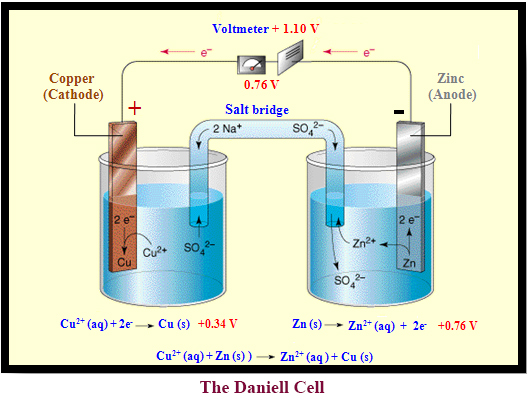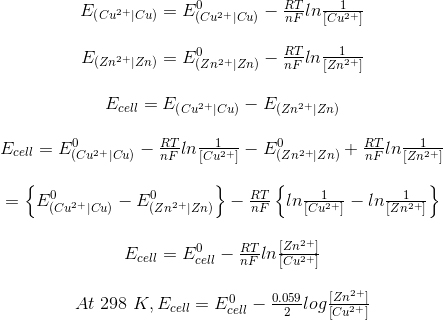Determination of EMF of a Cell

# Our Objective

Our objective is to study the variation of cell potential of Zn│Zn2+ ││Cu2+│Cu cell with change in the concentration of electrolytes (CuSO4 and ZnSO4) at room temperature.

# The Theory

## What is Electrochemistry?

Electrochemistry is the study of the interchange of chemical energy and electrical energy. The process of conversion of chemical energy into electrical energy is called electrochemical reaction and the reverse process is called electrolytic reaction or electrolysis. A device that produces electrical energy from a chemical reaction is called an electrochemical cell and devices that undergo chemical reaction with the aid of electricity are called electrolytic cells.

## Galvanic Cell

A galvanic cell is an important electrochemical cell. It is named after Luigi Galvani an Italian physicist. It is also called Voltaic cell, after an Italian physicist, Alessandro Volta. A galvanic cell generally consists of two different metal rods called electrodes. Each electrode is immersed in a solution containing its own ions and  these form a half cell. Each half cell is connected by a salt bridge, or separated by a porous membrane. The solutions in which the electrodes are immersed are called electrolytes.

The chemical reaction that takes place in a galvanic cell is the redox reaction. One electrode acts as anode in which oxidation takes place and the other acts as the cathode in which reduction takes place.  The best example of a galvanic cell is the Daniell cell.

## Daniell cell

The Daniell cell was invented by a British chemist, John Frederic Daniell. In the Daniell cell, copper and zinc electrodes are immersed in a solution of copper (II) sulfate (CuSO4 (aq)) and zinc (II) sulfate (ZnSO4 (aq)) respectively. The two half cells are connected through a salt bridge. Here zinc acts as anode and copper acts as cathode.

At the anode, zinc undergoes oxidation to form zinc ions and electrons.  The zinc ions pass into the solution. If the two electrodes are connected using an external wire, the electrons produced by the oxidation of zinc travel through the wire and enter into the copper cathode, where they reduce the copper ions present in the solution and form copper atoms that are deposited on the cathode.

The anodic reaction is represented as:The cathodic reaction is represented as:Total cell reaction is the sum of the two half cell reactions:## What is a Salt Bridge?

The salt bridge is usually an inverted U-tube filled with a concentrated solution of an inert electrolyte. The inert electrolyte is neither involved in any chemical change, nor does it react with the solutions in the two half cells.  Generally salts like, KCl, KNO3, NH4NO3 are used as the electrolyte.

## How is a Salt Bridge made?

To prepare salt bridge, agar-agar or gelatin is mixed with a hot concentrated solution of electrolyte and is filled in the U-tube. On cooling, the solution sets in the form of a gel inside the U-tube and thus prevents the inter mixing of the fluids. The two ends of the U-tube are then plugged with cotton wool to minimise diffusion.

## Significance of Salt Bridge

• Its main function is to prevent the potential difference that arise between the two solutions when they are in contact with each other. This potential difference is called the liquid junction potential.
• It completes the electrical circuit by connecting the electrolytes in the two half cells.
• It prevents the diffusion of solutions from one half cell to the other.
• It maintains the electrical neutrality of the solutions in the two half cells.

### How is the electrical neutrality of the solutions in the two half cells maintained using a salt bridge?

In the anodic half cell, there will be accumulation of positive charge when the positive ions that are formed pass into the solution. To maintain the electrical neutrality, salt bridge provides negative ions.

For example, in Daniell cell, zinc oxidizes at the anode and passes into the solutions as Zn2+ ions, so there will be accumulation of positive charge in the solution. To maintain the electrical neutrality of the solution, the salt bridge provides negative ions (may be NO3- or Cl-).

In the cathodic half cell, there will be accumulation of negative ions formed due to the reduction of positive ions. To maintain the electrical neutrality, salt bridge provides postive ions.

For example, in Daniell cell, Cu2+ ions from the CuSO4 solution is reduced by the electron formed by the oxidation of zinc, and deposited on the copper cathode. As a result, the concentration Cu2+ ions decreases in the solution and that of SO42- ions (sulphate ions) increases. So there will be an accumulation of negatively charged sulphate ions around the cathode. To maintain the electrical neutrality, salt bridge provides positive ions (may be, K+ or NH4+).

## Representation of an electrochemical cell: The cell notation

The following rules are followed for representing an electrochemical cell.

• Anode is written on the left hand side and cathode on the right hand side.
• The anodic cell is represented by writing the metal first and then the metal ions present in the electrolyte. These two are separated by a vertical line or a semicolon.

For example:• The molar concentration or activity of the solution is written in brackets after the formula of the ion.

For example:• The cathodic cell is represented by writing the metal ion first of the electrolyte solution and then the metal. Both are separated by vertical line or semicolon.

For example:• The molar concentration or activity of the solution is written in brackets after the formula of the ion.

For example:• Salt bridge is represented by two vertical lines.

So Daniell cell is represented as:If the concentration of both the electrolytes is 1 M, then the cell notation is:## Electrode potential

When a metal electrode is dipped in a solution containing its metal ions, a potential difference is developed at the metal solution interface. This potential difference is called the electrode potential.

For example, when a zinc rod is dipped in a solution containing Zn2+ ions, it oxidizes, and the Zn2+ ions pass from the zinc rod to the solution leaving excess of electrons at the zinc rod. Thus the zinc rod becomes negatively charged with respect to the solution and a potential difference is set up between the zinc rod and the solution. This potential difference is called the electrode potential of zinc. Similarly, when a copper rod is dipped in a solution containing Cu2+ ions, the Cu2+ ions gain electrons from the copper rod leaving positive charge on the copper rod. As a result a potential difference is set up between the copper rod and the solution and is called the electrode potential of copper.

In an electrochemical cell, the anode has a negative potential and cathode has a positive potential.The potential of each individual half cell cannot be measured. We can measure only the difference between the potential of the two half cells.

The potential of a half cell can be measured by connecting it with Standard Hydrogen Electrode (SHE). The standard electrode potential of a SHE is assumed to be zero.

The electrode potential at standard conditions such as 25°C temperature, 1 atm pressure, 1 M concentration of electrolyte, is called the standard electrode potential. It is denoted by the symbol E0. The electrodes are arranged in the increasing order of their standard reduction potential and are called electrochemical series.## Electromotive Force (emf) OR Cell Potential

The potential difference between the two electrodes in a galvanic cell is called a cell potential or emf of the cell. It is measured in volts.

emf of the cell = Potential of the half cell on the right hand side (Cathode) - Potential of the half cell on the left hand side (Anode)

For example, emf of Daniell cell,##Determination of the emf of a cell: The Nernst Equation

Nernst equation relates the equilibrium potential of an half cell with the standard electrode potential, temperature, activity and reaction quotients of the reacting species. It is named after the German physical chemist Walther Nernst.

Nernst showed that for the electrode reaction;, the electrode potential at any concentration measured with respect to SHE can be represented as:Where,is the electrode potential of the half cell,is the standard electrode potential,is the concentration of the metal,is the concentration of the metal ion,is the universal gas constant (8.314 J/K/mole),is the temperature in kelvin,is the number of electron involved in the reaction, andis the Faradays constant (96500 C/mole).

But the concentration of the solid M is taken as unity and substituting the values of R, F and T=298 K (Room Temperature), the above equation reduces to:## Calculation of emf of Daniell Cell

In Daniell cell, the electrode potentials of the half cells are written as:So Nernst equation is generally represented as:Where,is the activity (Concentration) of reduced species, andis the activity (Concentration) of oxidised species.

So the electrode potential of an electrochemical depends on the standard electrode potential, temperature, no. of electrons involved in the chemical reaction and the concentration of the reacting species.

# Learning Outcomes

• Students understand the terms, electrochemical cell, electrolytic cell, Daniell cell, salt bridge, EMF.
• Students acquire the skill to construct a Daniell cell.
• Students understand the significance of salt bridge.
• Students acquire the skill to calculate the electrode potential of a half cell and full cell using the Nernst equation.
• Students acquire the skill to measure the EMF of a cell by viewing animation & simulator.
• Students understand how the electrode potential of a given cell varies with the concentration of electrolytes in the anodic and cathodic half cell.